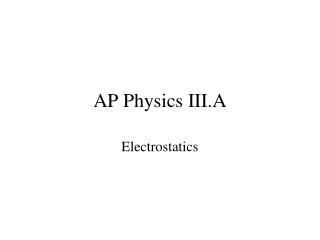DownloadDownload PresentationAP Physics III.A

# AP Physics III.A

Download Presentation## AP Physics III.A

- - - - - - - - - - - - - - - - - - - - - - - - - - - E N D - - - - - - - - - - - - - - - - - - - - - - - - - - -
##### Presentation Transcript

1. AP Physics III.A Electrostatics

2. 18.1 Origin of Electricity

3. 18.2 Charged Objects and Electric Force

4. Law of Conservation of Charge – during any one process, net electrical charge of an isolated system remains constant.

5. Ex. Two identical isolated conducting spheres, one with charge -6 μC and another with net charge +2 μC are allowed to touch. If the two spheres have the same net charge after touching, what is the net charge on each sphere?

6. Attractions and repulsions

7. 18.3 Conductors and Insulators

8. 18.4 Charging by Induction and Conduction (also known as, “I wish I had a decent electroscope”)

9. Charging by Conduction

10. Charging by Induction

11. Induced charge on an insulator

12. 18.5 (Charles De) Coulomb’s Law

13. “Hmm, this looks like something I’ve seen before”

14. Ex. An electron “orbits” the proton of a hydrogen atom at an average distance of 0.53 EE 10-10 m. What is the force that the proton exerts on the electron? What is the velocity of the electron for a circular orbit?

15. Ex. Two charges exert electrical force F on each other. If the magnitude of each charge is doubled and the distance between them is halved, what is the force F′ on each charge in terms of F?

16. Electric forces and vectors

17. Ex. Three Charges in a Line

18. Ex. Three Charges in a Plane

19. p. 552: 3-5, 7, 11, 15, 19, 21, 23, 79B7 • 3.4 EE -17 kg, mA > mB 79B7 • FBD b)

20. 18.6 Electric Field

21. A mass in a gravitational field

22. Charges experience an electrostatic force due to the presence of other charges

23. Force per Coulomb is the definition of an electric field (“show me the formula”)

24. An electric field is a vector that has a direction that the force exerts on a positive test charge.

25. Some examples

26. Ex. Find the electric force on a proton placed in an electric field of 2.0 EE 4 N/C that is directed along the positive x-axis.

27. Electric fields are vectors. The net electric field at a point in space can be determined by considering the contributions of each charged object and adding them together as vectors.

28. Electric field produced by a point charge

29. Ex. Electric Field Between Two Point Charges. Two point charges are separated by a distance of 0.100 m. One has a charge of –25.0 μC and the other 50.0 μC. a) What is the magnitude and direction of the electric field at point P between them 0.020 m from the negative charge? b) If an electron is placed at rest at P, what is the magnitude and direction of its initial acceleration?

30. Symmetry and the electric field.

31. 18.7 Electric Field Lines

32. Field lines around positive and negative charges

33. Field lines between plates of a capacitor.

34. Field lines between two dipoles

35. Field lines between two identical charges

36. p. 553: 25, 29, 31, 35-37; 81B3 • a) 182 N/C b) 312 N/C 81B3 • FBD b) E = 5800 N/C, FT = 0.058 N c) drawing

37. 19.1 Electric Potential Energy

38. Work done on a charge in a uniform electric field

39. Let’s clarify but not overemphasize the signs

40. 19.2 Electric Potential Difference

41. Let’s look at “gravitational potential” first

42. OK, now electric potential

43. So change in electric potential is . . .

44. Electric potential decreases or increases not because the field exerts any more or less force (the field is uniform – like gravity near the Earth’s surface). V changes because of distance. A charge released in the field, traveling a greater distance converts more of its Ue to K (like dropping an object from a greater height).

45. Everyday examples

46. Potential (and therefore potential difference) is scalar (this will simplify some things).

47. Summary • Electric potential energy – energy a charge has because of its potential in an electric field (so far the field is uniform) • Electric potential – electric potential energy per unit charge • Potential difference – change in electric potential